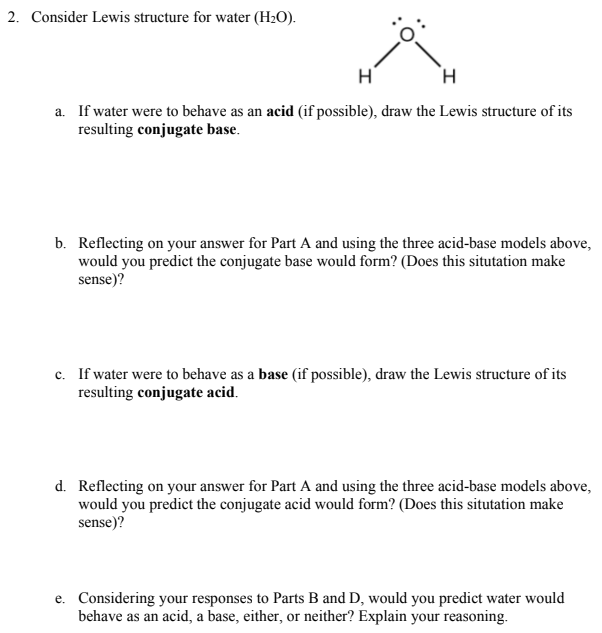# Consider Lewis structure for water (H2O). a. If water were to behave as an acid (if possible), draw the Lewis structure of its resulting conjugate base. b. Reflecting on your answer for Part A and using the three acid-base models above, would you predict the conjugate base would form? (Does this situation make sense)? c. If water were to behave as a base (if possible), draw the Lewis structure of its resulting conjugate acid. d. Reflecting on your answer for Part A and using the three acid-base models above, would you predict the conjugate acid would form? (Does this situation make sense)? e. Considering your responses to Parts B and D, would you predict water would behave as an acid, a base, either, or neither? Explain your reasoning.